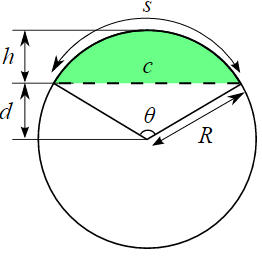Related Resources: math

### Circular Segment Equation and Calculator

Engineering Math

Circular Segment Equation and Calculator

Circular segment is a region of a circle which is "cut off" from the rest of the circle by a secant or a chord . More formally, a circular segment is a region of two-dimensional space that is bounded by an arc (of less than 180°) of a circle and by the chord connecting the endpoints of the arc.Where:

R = h + d = h / 2 + c2 / ( 8h )

Arc Length:

s = arcsin ( c / ( h + c2 / 4h ) ) ( h + c2 / 4h )

Chord Length: θ given in radians.

c = 2 R sin ( θ / 2 )

Angle:

θ = 2 arctan ( c / ( 2 d ) )

h = R ( 1 - cos ( θ / 2) )

Area:

The area A of the circular segment is equal to the area of the circular sector minus the area of the triangular portion. θ given in radians.

A = (R2 / 2 ) ( θ - sinθ )

A = ( R2 / 2 ) ( ( α Π ) / 180° - sinθ )

Central angle in degrees